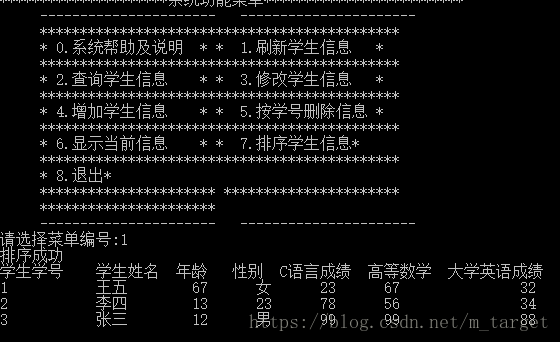﻿ C++使用文件实现学生信息管理系统_C 语言_脚本之家

# C++使用文件实现学生信息管理系统

更新时间：2021年01月25日 16:35:11   作者：m_target

C++实现文件实现学生信息管理系统，供大家参考，具体内容如下

### 功能如下：

• 成绩信息（c语言，大学英语，高等数学）
• 个人信息（姓名、性别、学号、年龄）

• 按学号查询
• 按名字查询

### 类的设计：

```class Score{

public:
float cLauage;//c语言成绩
float math;//高数成绩
float english;//英语成绩
};```

```class Student{
public :
char num;//学号
int age;//年龄
char name;//姓名
char sex;//性别
Score sc;
};```

```class Teacher{
public:
Student stu[N]; //N为50
void seek();//查找
void modify();//修改信息
void insert();//插入学生信息
void del();//删除学生
void display();//显示学生信息
void sorts();//按学号排序
void help();//帮助信息
void refresh();//刷新
};```

### 主要功能的实现

```//插入学生信息
void Teacher::insert(){

int j=n;
int m;//要增加的学生人数
cout<<"请输入待增加的学生数:"<<endl;
cin>>m;
do
{
Student stu;
cout<<"请输入第"<<j-n+1<<"个学生的学号"<<endl;//输入学生信息
cin>>stu.num;
cout<<"请输入第"<<j-n+1<<"个学生的姓名"<<endl;
cin>>stu.name;
cout<<"请输入第"<<j-n+1<<"个学生的年龄"<<endl;
cin>>stu.age;
cout<<"请输入第"<<j-n+1<<"个学生的性别"<<endl;
cin>>stu.sex;
cout<<"请输入第"<<j-n+1<<"个学生的C语言成绩"<<endl;
cin>>stu.sc.cLauage;
cout<<"请输入第"<<j-n+1<<"个学生的高数成绩"<<endl;
cin>>stu.sc.math;
cout<<"请输入第"<<j-n+1<<"个学生的英语成绩"<<endl;
cin>>stu.sc.english;
j++;
fstream file("student.txt", ios::out | ios::binary | ios::app);
file.write((char *)&stu, sizeof(Student));//把该学生对象写入文件
file.close();
}while(j<n+m);
n+=m;
cout<<"信息增加完毕"<<endl;
}```

```cout<<"请输入要查询的学生的学号"<<endl;
cin>>str;
fstream file("student.txt", ios::binary | ios::in);//打开文件
while(!file.eof())
{
Student stu;
if(strcmp(stu.num,str)==0) //判断从文件读出对象的学号信息是否和输入相等
{
flag=1;
cout<<"学生学号 学生姓名 年龄 性别 C语言成绩 高等数学 大学英语成绩"<<endl;
cout<<stu.num<<"\t "<<stu.name<<" \t"<<stu.age<<"\t"<<stu.sex<<"\t"<<stu.sc.cLauage<<" \t"<<stu.sc.math<<"\t\t "<<stu.sc.english<<endl;
break;
}
}
if(flag!=1)
cout<<"该学号不存在"<<endl;
file.close();```

```cout<<"请输入要查询的学生的姓名"<<endl;
cin>>str;
fstream file("student.txt", ios::binary | ios::in);
while(!file.eof())
{
Student stu;
if(strcmp(stu.name,str)==0)//判断从文件读出对象的姓名信息是否和输入相等
{
flag=1;
cout<<"学生学号 学生姓名 年龄 性别 C语言成绩 高等数学 大学英语成绩"<<endl;
cout<<stu.num<<"\t "<<stu.name<<" \t"<<stu.age<<"\t"<<stu.sex<<"\t"<<stu.sc.cLauage<<" \t"<<stu.sc.math<<"\t\t "<<stu.sc.english<<endl;
break;
}
}
if(flag!=1)
cout<<"该学生不存在"<<endl;
file.close();```

```void Teacher::display(){
int flag;
int t=0;
fstream file("student.txt", ios::binary | ios::in);
cout<<"学生学号 学生姓名 年龄 性别 C语言成绩 高等数学 大学英语成绩"<<endl;
while(!file.eof())
{
Student stu;
flag=1;
cout<<stu.num<<"\t "<<stu.name<<" \t"<<stu.age<<"\t"<<stu.sex<<"\t"<<stu.sc.cLauage<<" \t"<<stu.sc.math<<"\t\t "<<stu.sc.english<<endl;
}
if(flag!=1)
cout<<"无该学生"<<endl;
file.close();

}```

```void Teacher::modify(){
int item,age,score;//选择修改项,修改年龄
Student stu;
int flag;
char str;//暂时存修改内容
cout<<"请输入要查询的学生的学号"<<endl;
cin>>str;
fstream file("student.txt", ios::binary | ios::in);//打开文件存取学生对象的文件
while(!file.eof())
{
if(strcmp(stu.num,str)==0)//如果存在学生信息输出
{
flag=1;
cout<<"学生学号 学生姓名 年龄 性别 C语言成绩 高等数学 大学英语成绩"<<endl;
cout<<stu.num<<"\t "<<stu.name<<" \t"<<stu.age<<"\t"<<stu.sex<<"\t"<<stu.sc.cLauage<<" \t"<<stu.sc.math<<"\t\t "<<stu.sc.english<<endl;
break;
}
}
if(flag!=1)//如果不存在则提示无该学生
cout<<"该学生不存在"<<endl;
file.close();
printf("------------------\n");
printf("1.修改姓名\n");
printf("2.修改年龄\n");
printf("3.修改性别\n");
printf("4.修改C语言成绩\n");
printf("5.修改高等数学成绩\n");
printf("6.修改大学英语成绩\n");
printf("7.退出本菜单\n");
printf("------------------\n");
while(1)
{
printf("请选择子菜单编号:");
scanf("%d",&item);
switch(item)
{
case 1:
printf("请输入新的姓名:\n");
cin>>str;
strcpy(stu.name,str); break;//为学生姓名赋新值
case 2:
printf("请输入新的年龄:\n");
cin>>age;
stu.age=age;break;//为学生年龄赋新值
case 3:
printf("请输入新的性别:\n");
cin>>str;
strcpy(stu.sex,str); break;//为学生性别赋新值
case 4:
printf("请输入新的C语言成绩:\n");
cin>>score;
stu.sc.cLauage=score; break;//为学生成绩复新制
case 5:
printf("请输入新的数学成绩:\n");
cin>>score;
stu.sc.math=score; break;
case 6:
printf("请输入新的英语成绩:\n");
cin>>score;
stu.sc.english=score; break;
case 7:{
fstream file("student.txt", ios::out | ios::binary | ios::app);
cout<<"修改成功"<<endl;
file.write((char *)&stu, sizeof(Student));//把新的学生信息写入文件
file.close();
return;
}
default:printf("请在1-7之间选择\n");
}
}
}```

```void Teacher::del(){
vector<Student> va;
Student stu;//将读出的学生信息存入该对象
int flag;
char str;
cout<<"请输入要删除的学生的学号"<<endl;
cin>>str;
fstream file("student.txt", ios::binary | ios::in);//打开文件存取学生对象的文件
while(!file.eof())
{
if(strcmp(stu.num,str)==0)//如果存在学生信息输出
{
flag=1;
continue;
}
va.push_back(stu);//将非删除的学生存入容器
}
fstream file1("student.txt", ios::out | ios::trunc);
for (int i=0;i<va.size();i++)
{
file1.write((char *)&va[i], sizeof(Student));//将容器中的学生对象写入文件
}
cout<<"删除成功"<<endl;
file.close();//关闭文件
file1.close();
}```

```static bool myCompare(const Student& a1,const Student& a2)//自定义排序

{
return strcmp(a1.num,a2.num)<=0;//按学号从小到大排序
}

void Teacher::sorts(){
vector<Student> va;
Student stu;
fstream file("student.txt", ios::binary | ios::in);//打开文件存取学生对象的文件
while(!file.eof())
{
va.push_back(stu);//将学生对象存入容器
}
va.pop_back();
sort(va.begin(),va.end(),myCompare);//使用sort进行排序
fstream file1("student.txt", ios::out | ios::trunc);
for (int i=0;i<va.size();i++)
{
file1.write((char *)&va[i], sizeof(Student));//将排序好的写入文件
}
file.close();//关闭文件
file1.close();
}```

```//刷新
void Teacher::refresh(){
Teacher t;
t.sorts();
t.display();
}```

### 运行截图